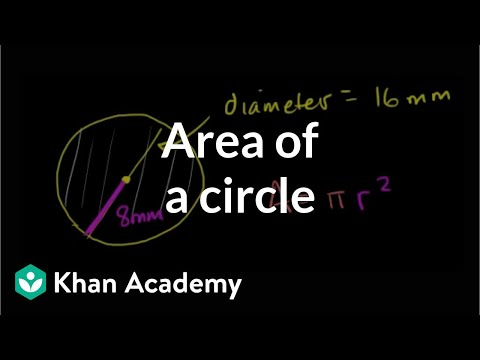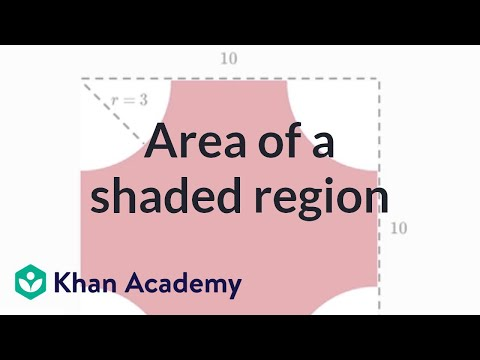Video

# Relating circumference and area (Full video)

Description: Sal uses formulas and a specific example to see how area and circumference relate. So we have a circle here, let's say that we know that its circumference is equal to six pi, I'll write it, units. Let's see if we can figure out, given that its circumference is 6 pi of these units, what is the area going to be equal to? And first, think about if you could figure out the area for this particular circle, and then let's see if we can come up with a formula for given any circumference, can we figure out the area and vice versa.

### Other videos you might be interested in### Area of a circle (Full video)